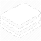DeepAI# Evaluation Metrics

## What are Evaluation Metrics?

Evaluation metrics are used to measure the quality of the statistical or machine learning model. Evaluating machine learning models or algorithms is essential for any project. There are many different types of evaluation metrics available to test a model. These include classification accuracy, logarithmic loss, confusion matrix, and others. Classification accuracy is the ratio of the number of correct predictions to the total number of input samples, which is usually what we refer to when we use the term accuracy. Logarithmic loss, also called log loss, works by penalizing the false classifications. A confusion matrix gives us a matrix as output and describes the complete performance of the model. There are other evaluation metrics that can be used that have not been listed. Evaluation metrics involves using a combination of these individual evaluation metrics to test a model or algorithm.

### Why is this Useful?

It is very important to use multiple evaluation metrics to evaluate your model. This is because a model may perform well using one measurement from one evaluation metric, but may perform poorly using another measurement from another evaluation metric. Using evaluation metrics are critical in ensuring that your model is operating correctly and optimally.

### Applications of Evaluation Metrics

• Statistical Analysis
• Machine Learning